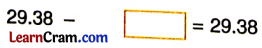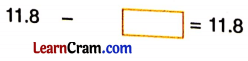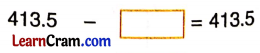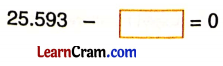# DAV Class 5 Maths Chapter 6 Worksheet 5 Solutions

The DAV Maths Book Class 5 Solutions and DAV Class 5 Maths Chapter 6 Worksheet 5 Solutions of Addition and Subtraction of Decimal Numbers offer comprehensive answers to textbook questions.

## DAV Class 5 Maths Ch 6 Worksheet 5 Solutions

Question 1.
Complete the following:
(a)Solution:
2.65

(b)Solution:
0(c)Solution:
0

(d)Solution:
19.5

(e)Solution:
0(f)Solution:
25.593

DAV Class 5 Maths Chapter 6 Worksheet 5 Notes

When we subtract zero from a decimal number, we get the decimal number itself.
e.g., (a) 13.176 – 0 = 13.176
(b) 13.001 – 0 = 13.001